# Weighted buffers in R

Had a request not so recently about implementing weighted buffer counts. The idea behind a weighted buffer is that instead of say counting the number of crimes that happen within 1,000 meters of a school, you want to give events that are closer to the school more weight.

There are two reasons you might want to do this for crime analysis:

• You want to measure the amount of crime around a location, but you rather have a weighted crime count, where crimes closer to the location have a greater weight than those further away.
• You want to measure attributes nearby a location (so things that predict crime), but give a higher weight to those closer to a location.

The second is actually more common in academic literature — see John Hipp’s Egohoods, or Liz Groff’s work on measuring nearby to bars, or Joel Caplan and using kernel density to estimate the effect of crime generators. Jerry Ratcliffe and colleagues work on the buffer intensity calculator is actually the motivation for the original request. So here are some quick code snippets in R to accomplish either. Here is the complete code and original data to replicate.

Here I use over 250,000 reported Part 1 crimes in DC from 08 through 2015, 173 school locations, and 21,506 street units (street segment midpoints and intersections) I constructed for various analyses in DC (all from open data sources) as examples.

## Example 1: Crime Buffer Intensities Around Schools

First, lets define where our data is located and read in the CSV files (don’t judge me setting the directory, I do not use RStudio!)

``````MyDir <- 'C:\\Users\\axw161530\\Dropbox\\Documents\\BLOG\\buffer_stuff_R\\Code' #Change to location on your machine!
setwd(MyDir)

Now there are several ways to do this, but here is the way I think will be most useful in general for folks in the crime analysis realm. Basically the workflow is this:

• For a given school, calculate the distance between all of the crime points and that school
• Apply whatever function to that distance to get your weight

For the function to the distance there are a bunch of choices (see Jerry’s buffer intensity I linked to previously for some example discussion). I’ve written previously about using the bi-square kernel. So I will illustrate with that.

Here is an example for the first school record in the dataset.

``````#Example for crimes around school, weighted by Bisquare kernel
BiSq_Fun <- function(dist,b){
ifelse(dist < b, ( 1 - (dist/b)^2 )^2, 0)
}

S1 <- t(SchoolLoc[1,2:3])
Dis <- sqrt( (CrimeData\$BLOCKXCOORD - S1)^2 + (CrimeData\$BLOCKYCOORD - S1)^2 )
Wgh <- sum( BiSq_Fun(Dis,b=2000) )``````

Then repeat that for all of the locations that you want the buffer intensities, and stuff it in the original `SchoolLoc` data frame. (Takes less than 30 seconds on my machine.)

``````SchoolLoc\$BufWeight <- -1 #Initialize field

#Takes about 30 seconds on my machine
for (i in 1:nrow(SchoolLoc)){
S <- t(SchoolLoc[i,2:3])
Dis <- sqrt( (CrimeData\$BLOCKXCOORD - S)^2 + (CrimeData\$BLOCKYCOORD - S)^2 )
SchoolLoc[i,'BufWeight'] <- sum( BiSq_Fun(Dis,b=2000) )
}``````

In this example there are 173 schools and 276,621 crimes. It is too big to create all of the pairwise comparisons at once (which will generate nearly 50 million records), but the looping isn’t too cumbersome and slow to worry about building a KDTree.

One thing to note about this technique is that if the buffers are large (or you have locations nearby one another), one crime can contribute to weighted crimes for multiple places.

## Example 2: Weighted School Counts for Street Units

To extend this idea to estimating attributes at places just essentially swaps out the crime locations with whatever you want to calculate, ala Liz Groff and her inverse distance weighted bars paper. I will show something alittle different though, in using the weights to create a weighted sum, which is related to John Hipp and Adam Boessen’s idea about Egohoods.

So here for every street unit I’ve created in DC, I want an estimate of the number of students nearby. I not only want to count the number of kids in attendance in schools nearby, but I also want to weight schools that are closer to the street unit by a higher amount.

So here I read in the street unit data. Also I do not have school attendance counts in this dataset, so I just simulate some numbers to illustrate.

``````StreetUnits <- read.csv('DC_StreetUnits.csv')
StreetUnits\$SchoolWeight <- -1 #Initialize school weight field

SchoolLoc\$StudentNum <- round(runif(nrow(SchoolLoc),100,2000)) ``````

Now it is very similar to the previous example, you just do a weighted sum of the attribute, instead of just counting up the weights. Here for illustration purposes I use a different weighting function, inverse distance weighting with a distance cut-off. (I figured this would need a better data management strategy to be timely, but this loop works quite fast as well, again under a minute on my machine.)

``````#Will use inverse distance weighting with cut-off instead of bi-square
Inv_CutOff <- function(dist,cut){
ifelse(dist < cut, 1/dist, 0)
}

for (i in 1:nrow(StreetUnits)){
SU <- t(StreetUnits[i,2:3])
Dis <- sqrt( (SchoolLoc\$XMeters - SU)^2 + (SchoolLoc\$YMeters - SU)^2 )
Weights <- Inv_CutOff(Dis,cut=8000)
StreetUnits[i,'SchoolWeight'] <- sum( Weights*SchoolLoc\$StudentNum )
}   ``````

The same idea could be used for other attributes, like sales volume for restaurants to get a measure of the business of the location (I think more recent work of John Hipp’s uses the number of employees).

Some attributes you may want to do the weighted mean instead of a weighted sum. For example, if you were using estimates of the proportion of residents in poverty, it makes more sense for this measure to be a spatially smoothed mean estimate than a sum. In this case it works exactly the same but you would replace `sum( Weights*SchoolLoc\$StudentNum )` with `sum( Weights*SchoolLoc\$StudentNum )/sum(Weights)`. (You could use the centroid of census block groups in place of the polygon data.)

## Some Wrap-Up

Using these buffer weights really just swaps out one arbitrary decision for data analysis (the buffer distance) with another (the distance weighting function). Although the weighting function is more complicated, I think it is probably closer to reality for quite a few applications.

Many of these different types of spatial estimates are all related to another (kernel density estimation, geographically weighted regression, kriging). So there are many different ways that you could go about making similar estimates. Not letting the perfect be the enemy of the good, I think what I show here will work quite well for many crime analysis applications.

# New working paper: Modeling the Spatial Patterns of Intra-Day Crime Trends

I have a new working paper out with Cory Haberman, Modeling the Spatial Patterns of Intra-Day Crime Trends. Below is the abstract:

Several prior studies have found that despite theoretical expectations otherwise, facilities (such as on-premise alcohol outlets) have consistent effects on crime regardless of time of the day (Bernasco et al., 2017; Haberman & Ratcliffe, 2015). We explain these results by failure to account for the regular background wave of crime, which results from ubiquitous patterns of human routine activities. Using eight years of data on assaults and robberies in Seattle (WA), we demonstrate the regularity of the within-day crime wave for all areas of the city. Then using models to predict when a crime will most likely occur, we demonstrate how schools and on-premise alcohol outlets cause bumps in the background wave at particular times of the day, such as when school dismisses. But those bumps dissipate quite rapidly in space, and are relatively small compared to the amplitude of the regular background wave of crime. Although facilities have theoretical times in which they should have a greater influence on crime patterns, they are situated within a community of other human activity uses, making it difficult to uniquely identify their effects separately from other aspects of the built environment.

And here is a joyplot showing the changes in the hour of day wave depending on how close robberies are to a public high school or middle school: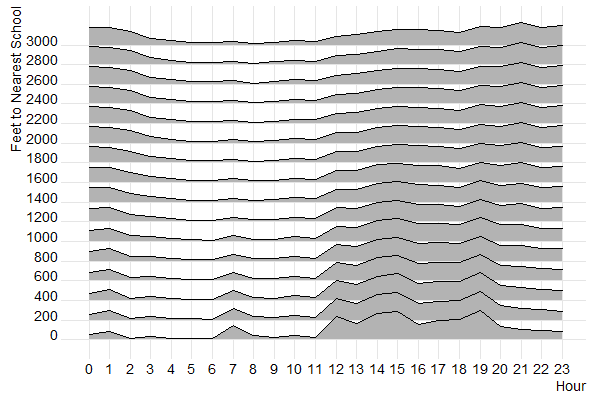You can see bumps very nearby schools at 7 am, then around noon and throughout the later afternoon, but are smoothed out when you get to around 2,000 feet away from schools.

The idea behind this paper is that several recent articles have not found much of a conditional relationship between crime generators and time of day. For example you would think bars only effect crime at nighttime when most people are at the bar, but several recent articles found the time of day does not make much of a difference (Bernasco et al., 2017; Haberman & Ratcliffe, 2015). We hypothesize this is because of the background wave of crime per hour of the day is much larger in magnitude than any local factor. An intuitive reason for this is that a place never has just a bar in isolation, there are other local land uses nearby that influence criminal patterns. You can see places nearby crime generators cause slight bumps in the background wave, but they are tiny compared to the overall amplitude of the general within day crime wave.

The article has a link to data and code to reproduce the findings. As always if you have feedback I am all ears.

# Testing the equality of coefficients – Same Independent, Different Dependent variables

As promised earlier, here is one example of testing coefficient equalities in SPSS, Stata, and R.

Here we have different dependent variables, but the same independent variables. This is taken from Dallas survey data (original data link, survey instrument link), and they asked about fear of crime, and split up the questions between fear of property victimization and violent victimization. Here I want to see if the effect of income is the same between the two. People in more poverty tend to be at higher risk of victimization, but you may also expect people with fewer items to steal to be less worried. Here I also control for the race and the age of the respondent.

The dataset has missing data, so I illustrate how to select out for complete case analysis, then I estimate the model. The fear of crime variables are coded as Likert items with a scale of 1-5, (higher values are more safe) but I predict them using linear regression (see the Stata code at the end though for combining ordinal logistic equations using `suest`). Race is of course nominal, and income and age are binned as well, but I treat the income bins as a linear effect. I pasted the codebook for all of the items at the end of the post.

These models with multiple dependent variables have different names, economists call them seemingly unrelated regression, psychologists will often just call them multivariate models, those familiar with structural equation modeling can get the same results by allowing residual covariances between the two outcomes — they will all result in the same coefficient estimates in the end.

# SPSS

In SPSS we can use the `GLM` procedure to estimate the model. Simultaneously we can specify particular contrasts to test whether the income coefficient is different for the two outcomes.

``````*Grab the online data.
SPSSINC GETURI DATA URI="https://dl.dropbox.com/s/r98nnidl5rnq5ni/MissingData_DallasSurv16.sav?dl=0" FILETYPE=SAV DATASET=MissData.

*Conducting complete case analysis.
COUNT MisComplete = Safety_Violent Safety_Prop Gender Race Income Age (MISSING).
COMPUTE CompleteCase = (MisComplete = 0).
FILTER BY CompleteCase.

*This treats the different income categories as a continuous variable.
*Can use GLM to estimate seemingly unrelated regression in SPSS and test.
*equality of the two coefficients.
GLM Safety_Violent Safety_Prop BY Race Age WITH Income
/DESIGN=Income Race Age
/PRINT PARAMETER
/LMATRIX Income 1
/MMATRIX ALL 1 -1.

FILTER OFF.  ``````

In the output you can see the coefficient estimates for the two equations. The income effect for violent crime is 0.168 (0.023) and for property crime is 0.114 (0.022).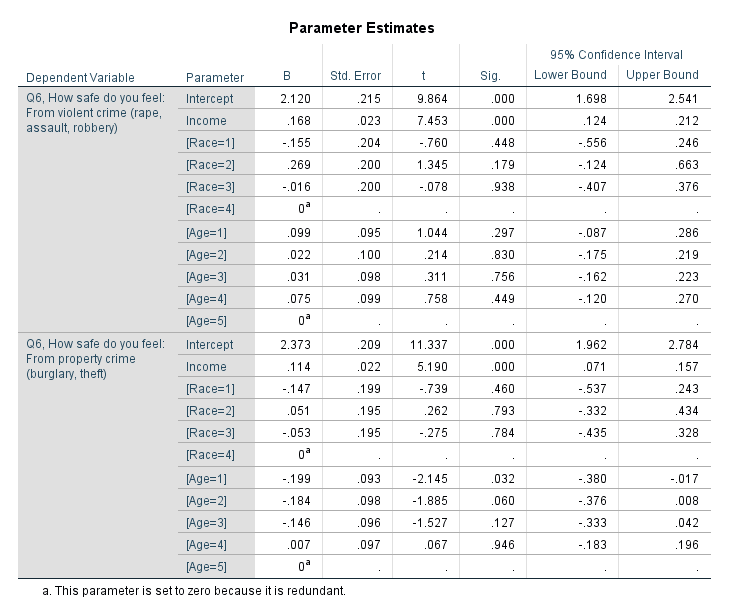And then you get a separate table for the contrast estimates.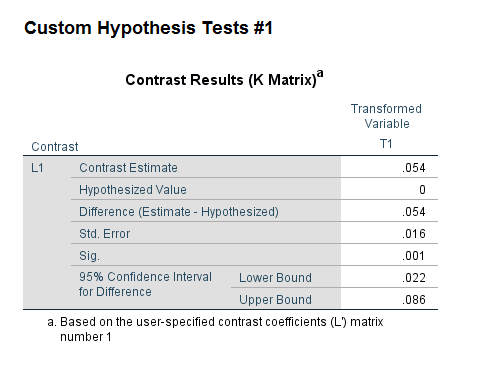You can see that the contrast estimate, 0.054, equals 0.168 – 0.114. The standard error in this output (0.016) takes into account the covariance between the two estimates. Here you would reject the null that the effects are equal across the two equations, and the effect of income is larger for violent crime. Because higher values on these Likert scales mean a person feels more safe, this is evidence that those with higher incomes are more likely to be fearful of property victimization, controlling for age and race.

Unfortunately the different matrix contrasts are not available in all the different types of regression models in SPSS. You may ask whether you can fit two separate regressions and do this same test. The answer is you can, but that makes assumptions about how the two models are independent — it is typically more efficient to estimate them at once, and here it allows you to have the software handle the Wald test instead of constructing it yourself.

# R

As I stated previously, seemingly unrelated regression is another name for these multivariate models. So we can use the R libraries `systemfit` to estimate our seemingly unrelated regression model, and then use the library `multcomp` to test the coefficient contrast. This does not result in the exact same coefficients as SPSS, but devilishly close. You can download the csv file of the data here.

``````library(systemfit) #for seemingly unrelated regression
library(multcomp)  #for hypothesis tests of models coefficients

names(SurvData) <- "Safety_Violent" #name gets messed up because of BOM

#Need to recode the missing values in R, use NA
NineMis <- c("Safety_Violent","Safety_Prop","Race","Income","Age")
#summary(SurvData[,NineMis])
for (i in NineMis){
SurvData[SurvData[,i]==9,i] <- NA
}

#Making a complete case dataset
SurvComplete <- SurvData[complete.cases(SurvData),NineMis]
#Now changing race and age to factor variables, keep income as linear
SurvComplete\$Race <- factor(SurvComplete\$Race, levels=c(1,2,3,4), labels=c("Black","White","Hispanic","Other"))
SurvComplete\$Age <- factor(SurvComplete\$Age, levels=1:5, labels=c("18-24","25-34","35-44","45-54","55+"))
summary(SurvComplete)

#now fitting seemingly unrelated regression
viol <- Safety_Violent ~ Income + Race + Age
prop <- Safety_Prop ~ Income + Race + Age
fitsur <- systemfit(list(violreg = viol, propreg= prop), data=SurvComplete, method="SUR")
summary(fitsur)

#testing whether income effect is equivalent for both models
viol_more_prop <- glht(fitsur,linfct = c("violreg_Income - propreg_Income = 0"))
summary(viol_more_prop) ``````

Here is a screenshot of the results then: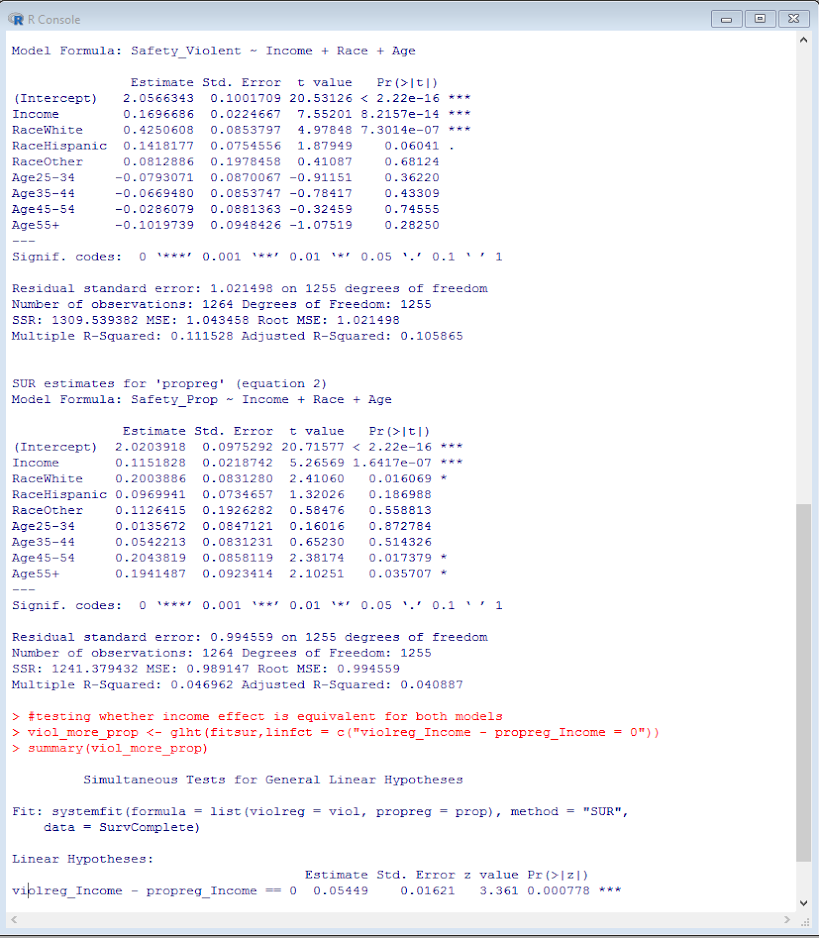This is also the same as estimating a structural equation model in which the residuals for the two regressions are allowed to covary. We can do that in R with the `lavaan` library.

``````library(lavaan)

#for this need to convert factors into dummy variables for lavaan
DumVars <- data.frame(model.matrix(~Race+Age-1,data=SurvComplete))
names(DumVars) <- c("Black","White","Hispanic","Other","Age2","Age3","Age4","Age5")

SurvComplete <- cbind(SurvComplete,DumVars)

model <- '
#regressions
Safety_Prop    ~ Income + Black + Hispanic + Other + Age2 + Age3 + Age4 + Age5
Safety_Violent ~ Income + Black + Hispanic + Other + Age2 + Age3 + Age4 + Age5
#residual covariances
Safety_Violent ~~ Safety_Prop
Safety_Violent ~~ Safety_Violent
Safety_Prop ~~ Safety_Prop
'

fit <- sem(model, data=SurvComplete)
summary(fit)``````

I’m not sure offhand though if there is an easy way to test the coefficient differences with a lavaan object, but we can do it manually by grabbing the variance and the covariances. You can then see that the differences and the standard errors are equal to the prior output provided by the `glht` function in `multcomp`.

``````#Grab the coefficients I want, and test the difference
PCov <- inspect(fit,what="vcov")
PEst <- inspect(fit,what="list")
Diff <- PEst[9,'est'] - PEst[1,'est']
SE <- sqrt( PEst[1,'se']^2 + PEst[9,'se']^2 - 2*PCov[9,1] )
Diff;SE``````

# Stata

In Stata we can replicate the same prior analyses. Here is some code to simply replicate the prior results, using Stata’s postestimation commands (additional examples using postestimation commands here). Again you can download the csv file used here. The results here are exactly the same as the R results.

``````*Load in the csv file
import delimited MissingData_DallasSurvey.csv, clear

*BOM problem again
rename ïsafety_violent safety_violent

*we need to specify the missing data fields.
*for Stata, set missing data to ".", not the named missing value types.
foreach i of varlist safety_violent-ownhome {
tab `i'
}

*dont specify district
mvdecode safety_violent-race income-age ownhome, mv(9=.)
mvdecode yearsdallas, mv(999=.)

*making a variable to identify the number of missing observations
egen miscomplete = rmiss(safety_violent safety_prop race income age)
tab miscomplete
*even though any individual question is small, in total it is around 20% of the cases

*lets conduct a complete case analysis
preserve
keep if miscomplete==0

*Now can estimate multivariate regression, same as GLM in SPSS
mvreg safety_violent safety_prop = income i.race i.age

*test income coefficient is equal across the two equations
lincom _b[safety_violent:income] - _b[safety_prop:income]

*same results as seemingly unrelated regression
sureg (safety_violent income i.race i.age)(safety_prop income i.race i.age)

*To use lincom it is the exact same code as with mvreg
lincom _b[safety_violent:income] - _b[safety_prop:income]

*with sem model
tabulate race, generate(r)
tabulate age, generate(a)
sem (safety_violent <- income r2 r3 r4 a2 a3 a4 a5)(safety_prop <- income r2 r3 r4 a2 a3 a4 a5), cov(e.safety_violent*e.safety_prop)

*can use the same as mvreg and sureg
lincom _b[safety_violent:income] - _b[safety_prop:income]``````

You will notice here it is the exact some post-estimation `lincom` command to test the coefficient equality across all three models. (Stata makes this the easiest of the three programs IMO.)

Stata also allows us to estimate seemingly unrelated regressions combining different generalized outcomes. Here I treat the outcome as ordinal, and then combine the models using seemingly unrelated regression.

``````*Combining generalized linear models with suest
ologit safety_violent income i.race i.age
est store viol

ologit safety_prop income i.race i.age
est store prop

suest viol prop

*lincom again!
lincom _b[viol_safety_violent:income] - _b[prop_safety_prop:income]``````

An application in spatial criminology is when you are estimating the effect of something on different crime types. If you are predicting the number of crimes in a spatial area, you might separate Poisson regression models for assaults and robberies — this is one way to estimate the models jointly. Cory Haberman and Jerry Ratcliffe have an application of this as well estimate the effect of different crime types at different times of day – e.g. the effect of bars in the afternoon versus the effect of bars at nighttime.

# Codebook

Here is the codebook for each of the variables in the database.

``````Safety_Violent
1   Very Unsafe
2   Unsafe
3   Neither Safe or Unsafe
4   Safe
5   Very Safe
9   Do not know or Missing
Safety_Prop
1   Very Unsafe
2   Unsafe
3   Neither Safe or Unsafe
4   Safe
5   Very Safe
9   Do not know or Missing
Gender
1   Male
2   Female
9   Missing
Race
1   Black
2   White
3   Hispanic
4   Other
9   Missing
Income
1   Less than 25k
2   25k to 50k
3   50k to 75k
4   75k to 100k
5   over 100k
9   Missing
Edu
1   Less than High School
2   High School
3   Some above High School
9   Missing
Age
1   18-24
2   25-34
3   35-44
4   45-54
5   55+
9   Missing
OwnHome
1   Own
2   Rent
9   Missing
YearsDallas
999 Missing``````

# Identifying near repeat crime strings in R or Python

People in criminology should be familiar with repeats or near-repeats for crimes such as robbery, burglaries, or shootings. An additional neat application of this idea though is to pull out strings of incidents that are within particular distance and time thresholds. See this example analysis by Haberman and Ratcliffe, The Predictive Policing Challenges of Near Repeat Armed Street Robberies. This is particularly useful to an analyst interested in crime linkage — to see if those particular strings of incidents are likely to be committed by the same offender.

Here I will show how to pluck out those near-repeat strings in R or Python. The general idea is to transform the incidents into a network, where two incidents are connected only if they meet the distance and time requirements. Then you can identify the connected components of the graph, and those are your strings of near-repeat events.

To follow along, here is the data and the code used in the analysis. I will be showing this on an example set of thefts from motor vehicles (aka burglaries from motor vehicles) in Dallas in 2015. In the end I take two different approaches to this problem — in R the solution will only work for smaller datasets (say n~5000 or less), but the python code should scale to much larger datasets.

# Near-repeat strings in R

The approach I take in R does the steps as follows:

1. compute the distance matrix for the spatial coordinates
2. convert this matrix to a set of 0’s and 1’s, 1’s correspond to if the distance is below the user specified distance threshold (call it S)
3. compute the distance matrix for the times
4. convert this matrix to a set of 0’1 and 1’s, 1’s correspond to if the distance is below the user specified time threshold (call it T)
5. use element-wise multiplication on the S and T matrices, call the result A, then set the diagonal of A to zero
6. A is now an adjacency matrix, which can be converted into a network
7. extract the connected components of that network

So here is an example of reading in the thefts from motor vehicle data, and defining my function, `NearStrings`, to grab the strings of incidents. Note you need to have the `igraph` R library installed for this code to work.

``````library(igraph)

MyDir <- "C:\\Users\\axw161530\\Dropbox\\Documents\\BLOG\\SourceNearRepeats"
setwd(MyDir)

summary(BMV)

#make a function
NearStrings <- function(data,id,x,y,time,DistThresh,TimeThresh){
library(igraph) #need igraph to identify connected components
MyData <- data
SpatDist <- as.matrix(dist(MyData[,c(x,y)])) < DistThresh  #1's for if under distance
TimeDist <-  as.matrix(dist(MyData[,time])) < TimeThresh #1's for if under time
AdjMat <- SpatDist * TimeDist #checking for both under distance and under time
diag(AdjMat) <- 0 #set the diagonal to zero
row.names(AdjMat) <- MyData[,id] #these are used as labels in igraph
colnames(AdjMat) <- MyData[,id] #ditto with row.names
CompInfo <- components(G) #assigning the connected components
return(data.frame(CompId=CompInfo\$membership,CompNum=CompInfo\$csize[CompInfo\$membership]))
}``````

So here is a quick example run on the first ten records. Note I have a field that is named `DateInt` in the csv, which is just the integer number of days since the first of the year. In R though if the dates are actual date objects you can submit them to the `dist` function though as well.

``````#Quick example with the first ten records
BMVSub <- BMV[1:10,]
ExpStrings <- NearStrings(data=BMVSub,id='incidentnu',x='xcoordinat',y='ycoordinat',time='DateInt',DistThresh=30000,TimeThresh=3)
ExpStrings``````

So here we can see this prints out:

``````> ExpStrings
CompId CompNum
000036-2015      1       3
000113-2015      2       4
000192-2015      2       4
000251-2015      1       3
000360-2015      2       4
000367-2015      3       1
000373-2015      4       2
000378-2015      4       2
000463-2015      2       4
000488-2015      1       3``````

The `CompId` field is a unique Id for every string of events. The `CompNum` field states how many events are within the string. So we have one string of events that contains 4 records in this subset.

Now this R function comes with a big caveat, it will not work on large datasets. I’d say your pushing it with 10,000 incidents. The issue is holding the distance matrices in memory. But if you can hold the matrices in memory this will still run quite fast. For 5,000 incidents it takes around ~15 seconds on my machine.

``````#Second example alittle larger, with the first 5000 records
BMVSub2 <- BMV[1:5000,]
BigStrings <- NearStrings(data=BMVSub2,id='incidentnu',x='xcoordinat',y='ycoordinat',time='DateInt',DistThresh=1000,TimeThresh=3)``````

The elements in the returned matrix will line up with the original dataset, so you can simply add those fields in, and do subsequent analysis (such as exporting back into a mapping program and digging into the strings).

``````#Add them into the original dataset
BMVSub2\$CompId <- BigStrings\$CompId
BMVSub2\$CompNum <- BigStrings\$CompNum   ``````

You can check out the number of chains of different sizes by using aggregate and table.

``````#Number of chains
table(aggregate(CompNum ~ CompId, data=BigStrings, FUN=max)\$CompNum)``````

This prints out:

``````   1    2    3    4    5    6    7    9
3814  405   77   27    3    1    1    1``````

So out of our first 1,000 incidents, using the distance threshold of 1,000 feet and the time threshold of 3 days, we have 3,814 isolates. Thefts from vehicles with no other incidents nearby. We have 405 chains of 2 incidents, 77 chains of 3 incidents, etc. You can pull out the 9 incident like this since there is only one chain that long:

``````#Look up the 9 incident
BMVSub2[BMVSub2\$CompNum == 9,]  ``````

Which prints out here:

``````> BMVSub2[BMVSub2\$CompNum == 9,]
incidentnu xcoordinat ycoordinat StartDate DateInt CompId CompNum
2094 043983-2015    2460500    7001459 2/25/2015      56   1842       9
2131 044632-2015    2460648    7000542 2/26/2015      57   1842       9
2156 045220-2015    2461162    7000079 2/27/2015      58   1842       9
2158 045382-2015    2460154    7000995 2/27/2015      58   1842       9
2210 046560-2015    2460985    7000089  3/1/2015      60   1842       9
2211 046566-2015    2460452    7001457  3/1/2015      60   1842       9
2260 047544-2015    2460154    7000995  3/2/2015      61   1842       9
2296 047904-2015    2460452    7001457  3/3/2015      62   1842       9
2337 048691-2015    2460794    7000298  3/4/2015      63   1842       9``````

Or you can look up a particular chain by its uniqueid. Here is an example of a 4-chain set.

``````> #Looking up a particular incident chains
> BMVSub2[BMVSub2\$CompId == 4321,]
incidentnu xcoordinat ycoordinat StartDate DateInt CompId CompNum
4987 108182-2015    2510037    6969603 5/14/2015     134   4321       4
4988 108183-2015    2510037    6969603 5/14/2015     134   4321       4
4989 108184-2015    2510037    6969603 5/14/2015     134   4321       4
4993 108249-2015    2510037    6969603 5/14/2015     134   4321       4``````

Again, only use this function on smaller crime datasets.

# Near-repeat strings in Python

Here I show how to go about a similar process in Python, but the algorithm does not calculate the whole distance matrix at once, so can handle much larger datasets. An additional note is that I exploit the fact that this list is sorted by dates. This makes it so I do not have to calculate all pair-wise distances – I will basically only compare distances within a moving window under the time threshold – this makes it easily scale to much larger datasets.

So first I use the `csv` python library to read in the data and assign it to a list with a set of nested tuples. Also you will need the `networkx` library to extract the connected components later on.

``````import networkx as nx
import csv
import math

dir = r'C:\Users\axw161530\Dropbox\Documents\BLOG\SourceNearRepeats'

BMV_tup = []
with open(dir + r'\TheftFromMV.csv') as f:
for row in z:
BMV_tup.append(tuple(row))``````

The `BMV_tup` list has the column headers, so I extract that row and then figure out where all the elements I need, such as the XY coordinates, the unique Id’s, and the time column are located in the nested tuples.

``````colnames = BMV_tup.pop(0)
print colnames
print BMV_tup[0:10]

xInd = colnames.index('xcoordinat')
yInd = colnames.index('ycoordinat')
dInd = colnames.index('DateInt')
IdInd = colnames.index('incidentnu')``````

Now the magic — here is my function to extract those near-repeat strings. Again, the list needs to be sorted by dates for this to work.

``````def NearStrings(CrimeData,idCol,xCol,yCol,tCol,DistThresh,TimeThresh):
G = nx.Graph()
n = len(CrimeData)
for i in range(n):
for j in range(i+1,n):
if (float(CrimeData[j][tCol]) - float(CrimeData[i][tCol])) > TimeThresh:
break
else:
xD = math.pow(float(CrimeData[j][xCol]) - float(CrimeData[i][xCol]),2)
yD = math.pow(float(CrimeData[j][yCol]) - float(CrimeData[i][yCol]),2)
d = math.sqrt(xD + yD)
if d < DistThresh:
comp = nx.connected_components(G)
finList = []
compId = 0
for i in comp:
compId += 1
for j in i:
finList.append((j,compId))
return finList``````

We can then do the same test on the first ten records that we did in R.

``print NearStrings(CrimeData=BMV_tup[0:10],idCol=IdInd,xCol=xInd,yCol=yInd,tCol=dInd,DistThresh=30000,TimeThresh=3)``

And this subsequently prints out:

``````[('000378-2015', 1), ('000373-2015', 1), ('000113-2015', 2), ('000463-2015', 2), ('000192-2015', 2), ('000360-2015', 2),
('000251-2015', 3), ('000488-2015', 3), ('000036-2015', 3)]``````

The component Id’s wont be in the same order as in R, but you can see we have the same results. E.g. the string with three incidents contains the Id’s 000251, 000488, and 000036. Note that this approach does not return isolates — incidents which have no nearby space-time examples.

Running this on the full dataset of over 14,000 incidents takes around 20 seconds on my machine.

``BigResults = NearStrings(CrimeData=BMV_tup,idCol=IdInd,xCol=xInd,yCol=yInd,tCol=dInd,DistThresh=1000,TimeThresh=3)``

And that should scale pretty well for really big cities and really big datasets. I will let someone who knows R better than me figure out workarounds to scale to bigger datasets in that language.

# Using the exact reference distribution for small sample Benford tests

I recently came across another potential application related to my testing small samples for randomness in day-of-week patterns. Testing digit frequencies for Benford’s law basically works on the same principles as my day-of-week bins. So here I will show an example in R.

First, download my functions here and save them to your local machine. The only library dependency for this code to work is the `partitions` library, so install that. Now for the code, you can source my functions and load the `partitions` library. The second part of the code shows how to generate the null distribution for Benford’s digits — the idea is that lower digits will have a higher probability of occurring.

``````#Example using small sample tests for Benfords law
library(partitions)
source("C:\\Users\\axw161530\\Dropbox\\PublicCode_Git\\ExactDist\\Exact_Dist.R")

f <- 1:9
p_fd <- log10(1 + (1/f)) #first digit probabilities``````

And so if you do `cbind(f,p_fd)` at the console it prints out:

``````      f       p_fd
[1,] 1 0.30103000
[2,] 2 0.17609126
[3,] 3 0.12493874
[4,] 4 0.09691001
[5,] 5 0.07918125
[6,] 6 0.06694679
[7,] 7 0.05799195
[8,] 8 0.05115252
[9,] 9 0.04575749``````

So we expect over 30% of the first digits to be 1’s, just under 18% to be 2’s, etc. To show how we can use this for small samples, I take an example of fraudulent checks from Mark Nigrini’s book, Digital Analysis using Benford’s law (I can’t find a google books link to this older one — it is the 2000 one published by Global Audit Press I took the numbers from).

``````#check data from Nigrini page 84
checks <- c(1927.48,
27902.31,
86241.90,
72117.46,
81321.75,
97473.96,
93249.11,
89658.17,
87776.89,
92105.83,
79949.16,
87602.93,
96879.27,
91806.47,
84991.67,
90831.83,
93766.67,
88338.72,
94639.49,
83709.28,
96412.21,
88432.86,
71552.16)

#extracting the first digits
fd <- substr(format(checks,trim=TRUE),1,1)
tot <- table(factor(fd, levels=paste(f)))``````

Now Nigrini says this is too small of a sample to perform actual statistical tests, so he just looks at it at face value. If you print out the `tot` object you will see that we have mostly upper values in the series, three 7’s, nine 8’s, and nine 9’s.

``````> tot

1 2 3 4 5 6 7 8 9
1 1 0 0 0 0 3 9 9 ``````

Now, my work on small samples for day-of-week crime sprees showed that given reasonably expected offender behavior, you only needed as few as 8 crimes to have pretty good power to test for randomness in the series. So given that I would expect the check series of 23 is not totally impossible to detect significant deviations from the null Benford’s distribution. But first we need to figure out if I can actually generate the exact distribution for 23 digits in 9 bins in memory.

``````m <- length(tot)
n <- sum(tot)
choose(m+n-1,m-1)``````

Which prints out just under 8 million, ` 7888725`. So we should be able to hold that in memory.

So now comes the actual test, and as my comment says this takes a few minutes for R to figure out — so feel free to go get a coffee.

``````#Takes a few minutes
resG <- SmallSampTest(d=tot,p=p_fd,type="G")
resG``````

Here I use the likelihood ratio G test instead of the more usual Chi-Square test, as I found that always had more power in the day-of-the-week paper. From our print out of `resG` we subsequently get:

``````> resG
Small Sample Test Object
Test Type is G
Statistic is 73.4505062784174
p-value is:  2.319579e-14
Data are:  1 1 0 0 0 0 3 9 9
Null probabilities are:  0.3 0.18 0.12 0.097 0.079 0.067 0.058 0.051 0.046
Total permutations are:  7888725 ``````

So since the p-value is incredibly small, we would reject the null that the first digit distribution of these checks follows Benford’s law. So on its face we can reject the null with this dataset, but it would take more investigation in general to give recommendations of how many observations in practice you would need before you can reasonably even use the small sample distribution. I have code that allows you to test the power given an alternative distribution in those functions, so for a quick and quite conservative test I see the power if the alternative distribution were uniform instead of Benford’s with our 23 observations. The idea is if people make up numbers uniformly instead of according to Benford’s law, what is the probability we would catch them with 23 observations. I label this as conservative, because I doubt people even do a good job of making them uniform — most number fudging cases will be much more egregious I imagine.

``````#power under null of equal probability
p_alt <- rep(1/9,9)
PowUni <- PowAlt(SST=resG,p_alt=p_alt) #again takes a few minutes
PowUni``````

So based on that we get a power estimate of:

``````> PowUni
Power for Small Sample Test
Test statistic is: G
Power is: 0.5276224
Null is: 0.3 0.18 0.12 0.097 0.079 0.067 0.058 0.051 0.046
Alt is: 0.11 0.11 0.11 0.11 0.11 0.11 0.11 0.11 0.11
Alpha is: 0.05
Number of Bins: 9
Number of Observations: 23 ``````

So the power is only 0.5 in this example. Since you want to aim for power of ~0.80 or higher, this shows that you are not likely to uncover more subtle patterns of manipulation with this few of observations. The power would go up for more realistic deviations though — so I don’t think this idea is totally dead in the water.

If you are like me and find it annoying to wait for a few minutes to get the results, a quick and dirty way to make the test go faster is to collapse bins that have zero observations. So our first digits have zero observations in the 3,4,5 and 6 bins. So I collapse those and do the test with only 6 bins, which makes the results return in around ~10 seconds instead of a few minutes. Note that collapsing bins is better suited for the G or the Chi-Square test, because the KS test or Kuiper’s test the order of the observations matter.

``````#Smaller subset
UpProb <- c(p_fd[c(1,2,7,8,9)],sum(p_fd[c(3,4,5,6)]))

resSmall``````

When you do this for the G and the Chi-square test you will get the same test statistics, but in this example the p-value is larger (but is still quite small).

``````> resSmall
Small Sample Test Object
Test Type is G
Statistic is 73.4505062784174
p-value is:  1.527991e-15
Data are:  1 1 3 9 9 0
Null probabilities are:  0.3 0.18 0.058 0.051 0.046 0.37
Total permutations are:  98280  ``````

I can’t say for sure the behavior of the tests when collapsing categories, but I think it is reasonable offhand, especially if you have some substantive reason to collapse them before looking at the data.

In practice, they way I expect this would work is not just for testing one individual, but as a way to prioritize audits of many individuals. Say you had a large company, and you wanted to check the invoices for 1,000’s of managers, but each manager may only have 20 some invoices. You would compute this test for each manager then, and then subsequently rank them by the p-values (or do some correction like the false-discovery-rate) for further scrutiny. That takes a bit more work to code that up efficiently than what I have here though. Like I may pre-compute the exact CDF’s for each test statistic, aggregate them alittle so they fit in memory, and then check the test against the relevant CDF.

But feel free to bug me if you want to use this idea in your own work and want some help implementing it.

For some additional examples, here is some code to get the second digit expected probabilities:

``````#second digit probabilities
s <- 0:9
x <- expand.grid(f*10,s)
x\$end <- log10(1 + (1/(x[,1]+x[,2])))
p_sd <- aggregate(end ~ Var2, data=x, sum)
p_sd``````

which are expected to be much more uniform than the first digits:

``````> p_sd
Var2        end
1     0 0.11967927
2     1 0.11389010
3     2 0.10882150
4     3 0.10432956
5     4 0.10030820
6     5 0.09667724
7     6 0.09337474
8     7 0.09035199
9     8 0.08757005
10    9 0.08499735``````

And we can subsequently also test the check sample for deviation from Benford’s law in the second digits. Here I show an example of using the exact distribution for the Kolmogorov-Smirnov test. (There may be reasonable arguments for using Kuiper’s test with digits as well, but for both the KS and the Kuiper’s V you need to make sure the bins are in the correct order to conduct those tests.) To speed up the computation I only test the first 18 checks.

``````#second digits test for sample checks, but with smaller subset
sd <- substr(format(checks[1:18],trim=TRUE),2,2)
tot_sd <- table(factor(sd, levels=paste(s)))
resK_2 <- SmallSampTest(d=tot_sd,p=p_sd,type="KS")
resK_2``````

And the test results are:

``````> resK_2
Small Sample Test Object
Test Type is KS
Statistic is 0.222222222222222
p-value is:  0.7603276
Data are:  1 2 2 2 1 0 2 4 1 3
Null probabilities are:  0.12 0.11 0.11 0.1 0.1 0.097 0.093 0.09 0.088 0.085
Total permutations are:  4686825 ``````

So for the second digits of our checks we would fail to reject the null that the data follow Benford’s distribution. To test the full 23 checks would generate over 28 million permutations – will update based on how long that takes.

The final example I will give is with another small example dataset — the last 12 purchases on my credit card.

``````#My last 12 purchases on credit card
purch <- c( 72.00,
328.36,
11.57,
90.80,
21.47,
7.31,
9.99,
2.78,
10.17,
2.96,
27.92,
14.49)
#artificial numbers, 72.00 is parking at DFW, 9.99 is Netflix   ``````

In reality, digits can deviate from Benford’s law for reasons that do not have to do with fraud — such as artificial constraints to the system. But, when I test this set of values, I fail to reject the null that they follow Benford’s distribution,

``````fdP <- substr(format(purch,trim=TRUE),1,1)
totP <- table(factor(fdP, levels=paste(f)))

resG_P <- SmallSampTest(d=totP,p=p_fd,type="G")
resG_P``````

So for a quick test the first digits of my credit card purchases do approximately follow Benford’s law.

``````> resG_P
Small Sample Test Object
Test Type is G
Statistic is 12.5740089945434
p-value is:  0.1469451
Data are:  3 4 1 0 0 0 2 0 2
Null probabilities are:  0.3 0.18 0.12 0.097 0.079 0.067 0.058 0.051 0.046
Total permutations are:  125970  ``````

# Some inverse distance weighting hacks – using R and spatstat

For a recent project I was mapping survey responses to attitudes towards the police, and I wanted to make a map of those responses. The typical default to accomplish this is inverse distance weighting. For those familiar with hot spot maps of crime, this is similar in that is produces a smooth isarithmic map, but instead of being a density it predicts values. For my project I wanted to explore two different things; 1) estimating the variance of the IDW estimate, and 2) explore different weighting schemes besides the default inverse distance. The R code for my functions and data for analysis can be downloaded here.

# What is inverse distance weighting?

Since this isn’t typical fodder for social scientists, I will present a simple example to illustrate.

Imagine you are a farmer and want to know where to plant corn vs. soy beans, and are using the nitrogen content of the soil to determine that. You take various samples from a field and measure the nitrogen content, but you want predictions for the areas you did not sample. So say we have four measures at various points in the field.

``````Nit     X   Y
1.2     0   0
2.1     0   5
2.6    10   2
1.5     6   5``````

From this lets say we want to estimate average nitrogen content at the center, 5 and 5. Inverse distance weighting is just as the name says, the weight to estimate the average nitrogen content at the center is based on the distance between the sample point and the center. Most often people use the distance squared as the weight. So from this we have as the weights.

``````Nit     X   Y Weight
1.2     0   0   1/50
2.1     0   5   1/25
2.6    10   2   1/34
1.5     6   5   1/ 1``````

You can see the last row is the closest point, so gets the largest weight. The weighted average of nitrogen for the 5,5 point ends up being ~1.55.

For inverse distance weighted maps, one then makes a series of weighted estimates at a regular grid over the study space. So not just an estimate at 5,5, but also 5,4|5,3|5,2 etc. And then you have a regular grid of values you can plot.

# Example – Street Clean Scores in LA

An ok example to demonstrate this is an LA database rating streets based on their cleanliness. Some might quibble about it only makes sense to estimate street cleanliness values on streets, but I think it is ok for exploratory data analysis. Just visualizing the streets is very hard given their small width and irregularity.

So to follow along, first I load all the libraries I will be using, then set my working directory, and finally import my updated inverse distance weighted hacked functions I will be using.

``````library(spatstat)
library(inline)
library(rgdal)
library(maptools)
library(ncf)

MyDir <- "C:\\Users\\axw161530\\Dropbox\\Documents\\BLOG\\IDW_Variance_Bisquare\\ExampleAnalysis"
setwd(MyDir)

#My updated idw functions
source("IDW_Var_Functions.R")``````

Next we need to create an point pattern object spatstat can work with, so we import our street scores that contain an X and Y coordinate for the midpoint of the street segment, as well as the boundary of the city of Los Angeles. Then we can create a marked point pattern. For reference, the street scores can range from 0 (clean) to a max of 3 (dirty).

``````CleanStreets <- read.csv("StreetScores.csv",header=TRUE)
summary(CleanStreets)

#create Spatstat object and window
LA_Win <- as.owin(BorderLA)
LA_StreetPP <- ppp(CleanStreets\$XMidPoint,CleanStreets\$YMidPoint, window=LA_Win, marks=CleanStreets\$StreetScor)``````

Now we can estimate a smooth inverse distance weighted map by calling my new function, `idw2`. This returns both the original weighted mean (equivalent to the original spatstat `idw` argument), but also returns the variance. Here I plot them side by side (see the end of the blog post on how I calculate the variance). The weighted mean is on the left, and the variance estimate is on the right. For the functions the rat image is the weighted mean, and the var image is the weighted variance.

``````#Typical inverse distance weighted estimate
idw_res <- idw2(LA_StreetPP) #only takes a minute
par(mfrow=c(1,2))
plot(idw_res\$rat) #this is the weighted mean
plot(idw_res\$var) #this is the weighted variance``````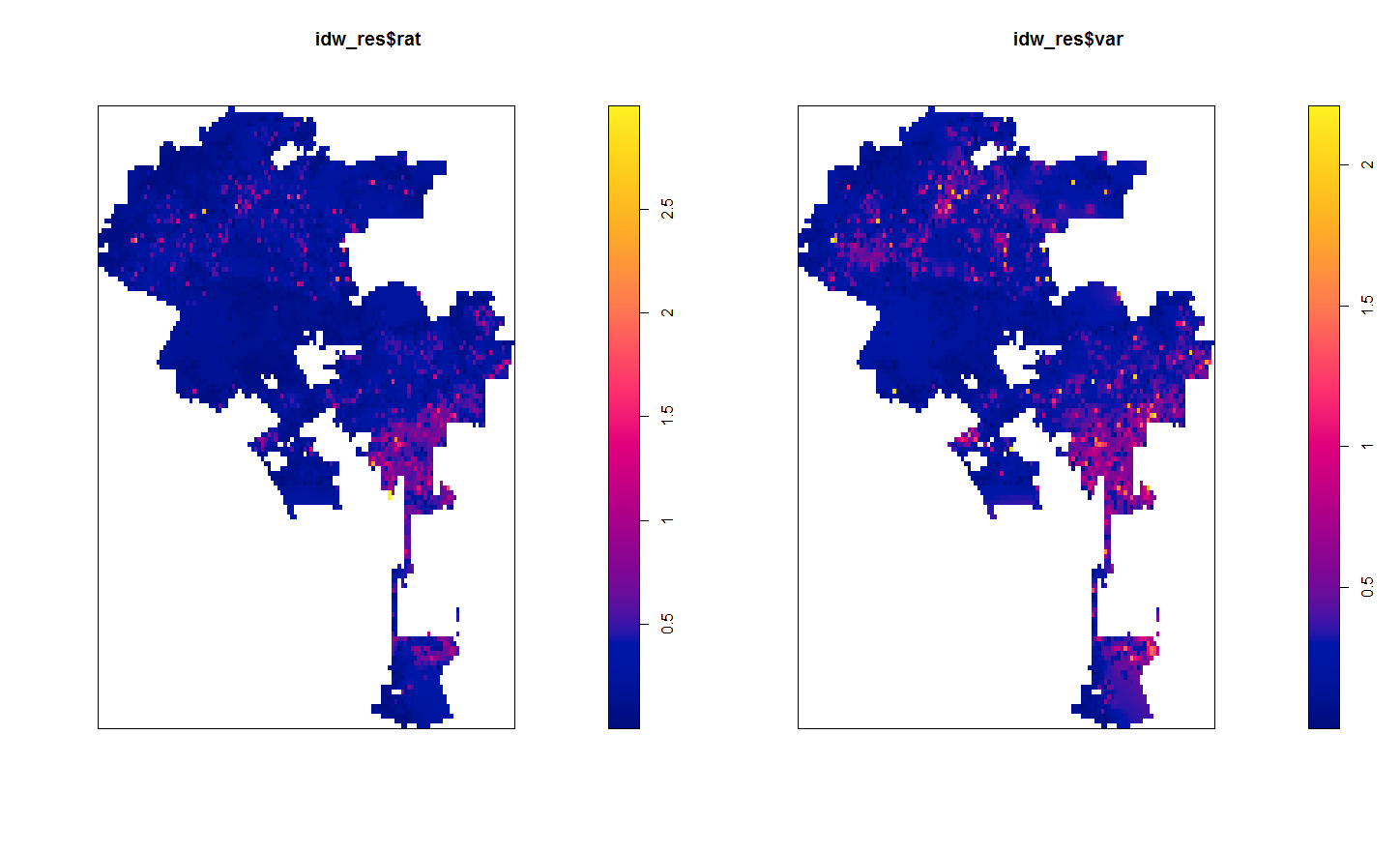So contrary to expectations, this does not provide a very smooth map. It is quite rough. This is partially because social science data is not going to be as regular as natural science measurements. In spatial stats jargon street to street measures will have a large nugget – a clean street can be right next to a dirty one.

Here the default is using inverse distance squared – what if we just use inverse distance though?

``````#Inverse distance (linear)
idw_Lin <- idw2(LA_StreetPP, power=1)
plot(idw_Lin\$rat)
plot(idw_Lin\$var)``````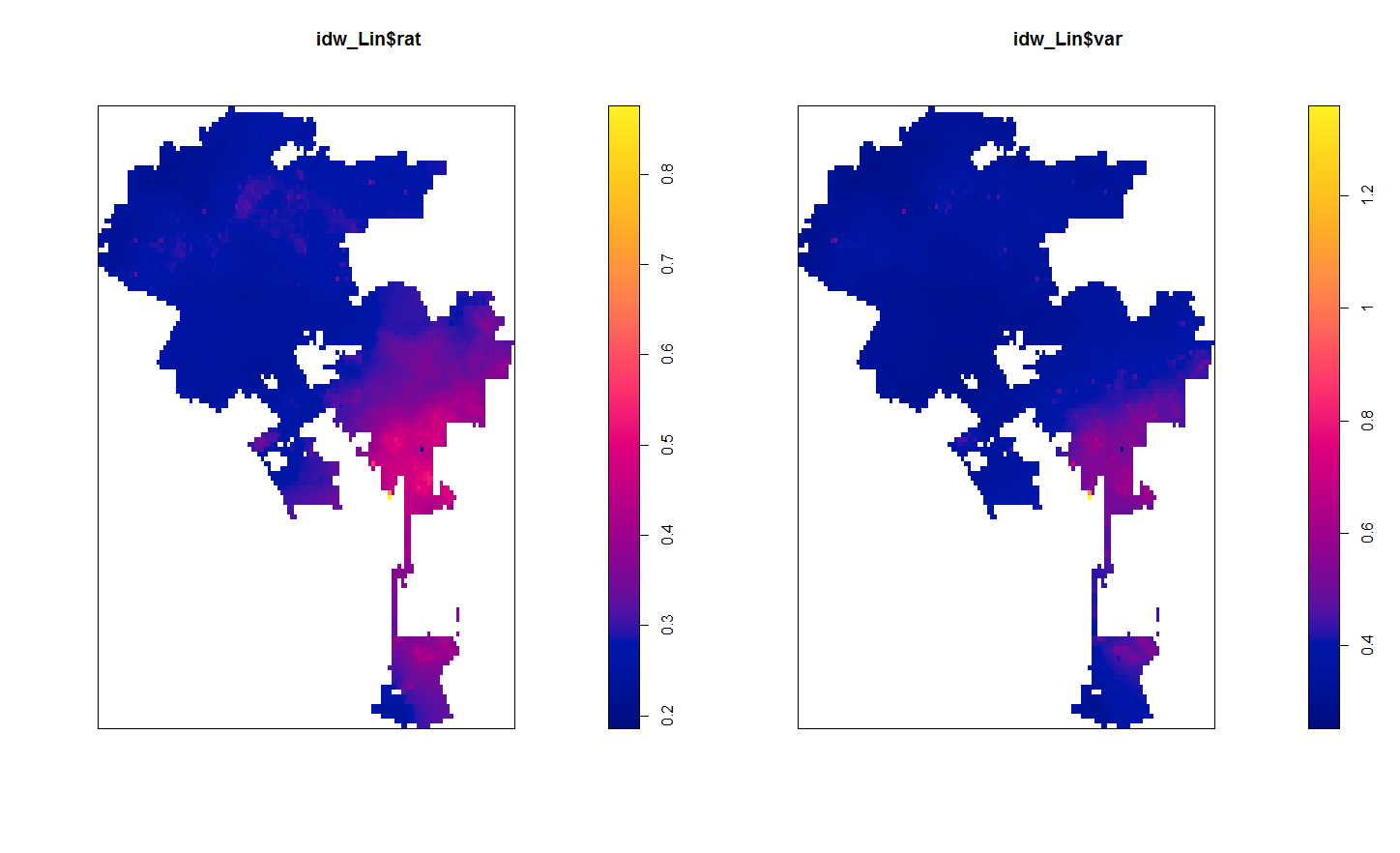This is smoothed out a little more. There is essentially one dirty spot in the central eastern part of the city (I don’t know anything about LA neighborhoods). Compared to the first set of maps, the dirty streets in the northern mass of the city are basically entirely smoothed out, whereas before you could at least see little spikes.

So I was wondering if there could maybe be better weights we could choose to smooth out the data a little better. One I have used in a few recent projects is the bisquare kernel, which I was introduced by the geographically weighted regression folks. The bisquare kernel weight equals `[1 - (d/b)^2]^2`, when `d < b` and zero otherwise. Here `d` is the distance, and `b` is a user chosen distance threshold. We can make a plot to illustrate the difference in weight functions, here using a bisquare kernel distance of 2000 meters.

``````#example weight functions over 3000 meters
dist <- 1:3000
idw1 <- 1/dist
idw2 <- 1/(dist^2)
b <- 2000
bisq <- ifelse(dist < b, ( 1 - (dist/b)^2 )^2, 0)
plot(dist,idw1,type='l')
lines(dist,idw2,col='red')
lines(dist,bisq,col='blue')``````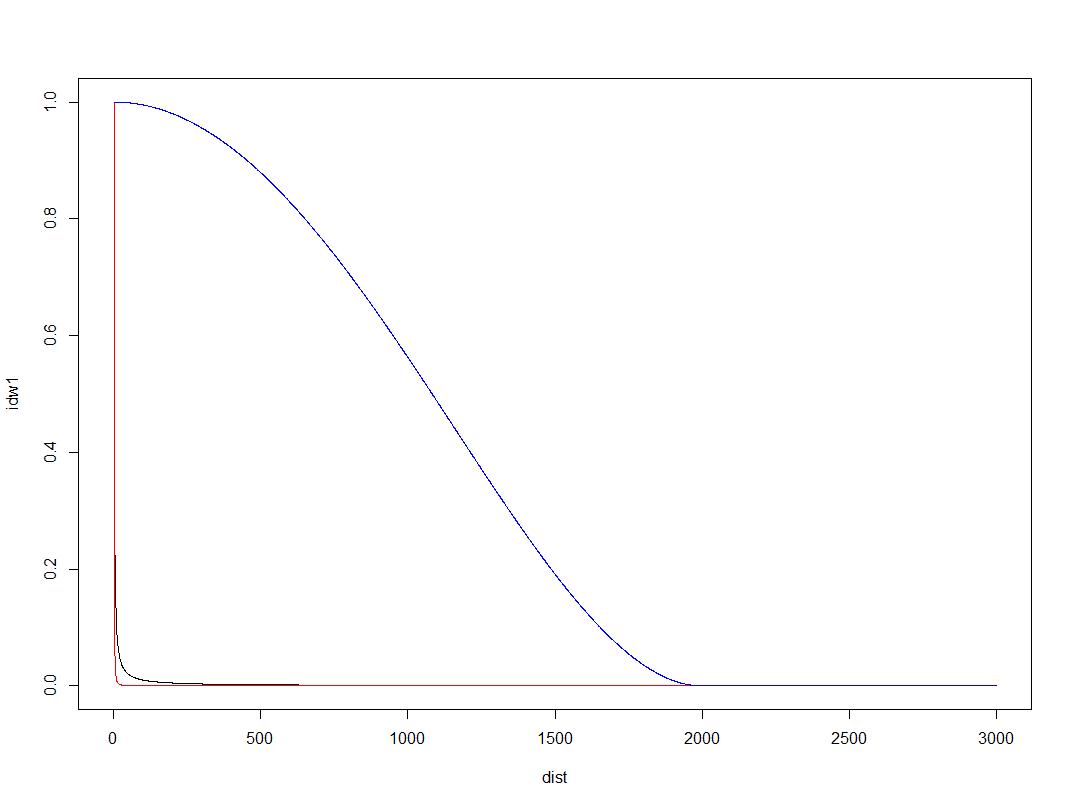Here you can see both of the inverse distance weighted lines trail to zero almost immediately, whereas the bisquare kernel trails off much more slowly. So lets check out our maps using a bisquare kernel with the distance threshold set to 2000 meters. The `biSqW` function is equivalent to the original spatstat `idw` function, but uses the bisquare kernel and returns the variance estimate as well. You just need to pass it a distance threshold for the `b_dist` parameter.

``````#BiSquare weighting, 2000 meter distance
LA_bS_w <- biSqW(LA_StreetPP, b_dist=2000)
plot(LA_bS_w\$rat)
plot(LA_bS_w\$var)``````Here we get a map that looks more like a typical hot spot kernel density map. We can see some of the broader trends in the northern part of the city, and even see a really dirty hot spot I did not previously notice in the northeastern peninsula.

The 2,000 meter distance threshold was just ad-hoc though. How large or small should it be? A quick check of the spatial correlogram is one way to make it slightly more objective. Here I use the `correlog` function in the `ncf` package to estimate this. I subsample the data first (I presume it has a call to `dist` somewhere).

``````#correleogram, random sample, it is too big
subSamp <- CleanStreets[sample(nrow(CleanStreets), 3000), ]
fit <- correlog(x=subSamp\$XMidPoint,y=subSamp\$YMidPoint,z=subSamp\$StreetScor, increment=100, resamp=0, quiet=TRUE)
plot(fit)``````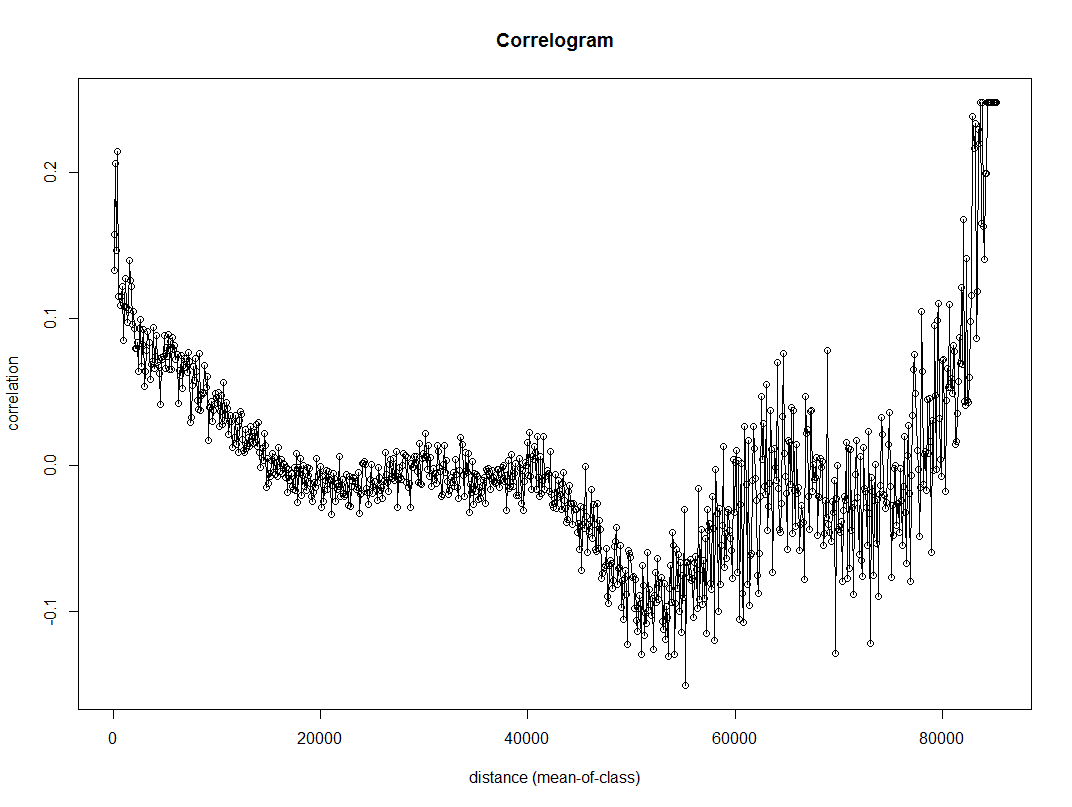Here we can see points very nearby each other have a correlation of 0.2, and then this trails off into zero before 20 kilometers (the distances here are in meters). FYI the rising back up in correlation for very large distances often occurs for data that have broader spatial trends.

So lets try out a bisquare kernel with a distance threshold of 10 kilometers.

``````#BiSquare weighting, 10000 meter distance
LA_bS_w <- biSqW(LA_StreetPP, b_dist=10000)
plot(LA_bS_w\$rat)
plot(LA_bS_w\$var)``````That is now a bit oversmoothed. But it allows a nicer range of potential values, as oppossed to simply sticking with the inverse distance weighting.

# A few notes on the variance of IDW

So I hacked the `idw` function in the spatstat package to return the variance of the estimate as well as the actual weighted mean. This involved going into the C function, so I use the `inline` package to create my own version. Ditto for creating the maps using the bisquare weights instead of inverse distance weighting. To quick see those functions here is the R code.

Given some harassment on Crossvalidated by Mark Stone, I also updated the algorithm to be a more numerically safe one, both for the weighted mean and the weighted variance. Note though that that Wikipedia article has a special definition for the variance. The correct Bessel correction for weighted data though (in this case) is the sum of the weights (V1) minus the sum of square of the weights (V2) divided by V1. Here I just divide by V1, but that could easily be changed (not sure if in the sum of squares I need to worry about underflow). I.e. change the line `MAT(var, ix, iy, Ny) = m2 / sumw;` to `MAT(var, ix, iy, Ny) = m2 / (sumw - sumw/sumw2);` in the various C calls.

Someone should also probably write in a check to prevent distances of zero. Maybe by capping the weights to never be above a certain value, although that is not trivial what the default top value should be. (If you have data on the unit square weights above 1 would occur quite regularly, but for a large city like this projected in meters capping the weight at 1 would be fine.)

In general these variance maps did not behave like I expected them to, either with this or other data. When using Bessel’s correction they tended to look even weirder. So I would need to explore some more before I go and recommend them. Probably should not waste more time on this though, and just fit an actual kriging model though to produce the standard error of the estimates.

I’ve written quite a few blog posts over the years, and it is getting to be hard for me to keep all of them orderly. So I recently created a page with a simple table of contents with code snippets broken down into categories. There are also a few extra SPSS macros in there that I have not written blog posts about.

Every now and then I see a broken link and update it, but I know there are more I am missing. So just send an email if I have a link to some of my code and it is currently broken.

# The spatial clustering of hits-vs-misses in shootings using Ripley’s K

My article, Replicating Group-Based Trajectory Models of Crime at Micro-Places in Albany, NY was recently published online first at the Journal of Quantitative Criminology (here is a pre-print version, and you can get my JQC offprint for the next few weeks).

Part of the reason I blog is to show how to replicate some of my work. I have previously shown how to generate the group based trajectory models (1,2), and here I will illustrate how to replicate some of the point pattern analysis I conducted in that paper.

A regular task for an analyst is to evaluate spatial clustering. A technique I use in that article is to use Ripley’s K statistic to evaluate clustering between different types of events, or what spatial statistics jargon calls a marked point pattern. I figured I would illustrate how to do this on some example point patterns of shootings, so analysts could replicate for other situations. The way crime data is collected and geocoded makes generating the correct reference distributions for the tests different than if the points could occur anywhere in the study region.

An example I am going to apply are shooting incidents that have marks of whether they hit the intended victim or missed. One theory in criminology is that murder is simply the extension of general violence — with the difference in aggravated assault versus murder often being happenstance. One instance this appears to be the case from my observations are shootings. It seems pure luck whether an individual gets hit or the bullets miss everyone. Here I will see if there appear to be any spatial patterning in shots fired with a victim hit. That is, we know that shootings themselves are clustered, but within those clusters of shootings are the locations of hits-and-misses further clustered or are they random?

A hypothetical process in which clustering of shooting hits can occur is if some shootings are meant to simply scare individuals vs. being targeted at people on the street. It could also occur if there are different tactics, like drive-bys vs. being on foot. This could occur if say one area had many shootings related to drug deals gone wrong, vs. another area that had gang retaliation drive by shootings. If they are non-random, the police may consider different interventions in different places – probably focusing on locations where people are more likely to be injured from the shooting. If they are random though, there is nothing special about analyzing shootings with a person hit versus shootings that missed everyone – you might as well analyze and develop strategy for all shootings.

So to start we will be using the R statistical package and the spatstat library. To follow along I made a set of fake shooting data in a csv file. So first load up R, I then change the working directory to where my csv file is located, then I read in the csv into an R data frame.

``````library(spatstat)

#change R directory to where your file is
MyDir <- "C:\\Users\\andrew.wheeler\\Dropbox\\Documents\\BLOG\\R_shootingVic\\R_Code"
setwd(MyDir)

The file subsequently has four fields, ID, X & Y coordinates, and a Vic column with 0’s and 1’s. Next to conduct the spatial analysis we are going to convert this data into an object that the spatstat library can work with. To do that we need to create a study window. Typically you would have the outline of the city, but for this analysis the window won’t matter, so here I make a window that is just slightly larger than the bounding box of the data.

``````#create ppp file, window does not matter for permuation test
StudyWin <- owin(xrange=c(min(ShootData\$X)-0.01,max(ShootData\$X)+0.01),yrange=c(min(ShootData\$Y)-0.01,max(ShootData\$Y)+0.01))
Shoot_ppp <- ppp(ShootData\$X, ShootData\$Y, window=StudyWin, marks=as.factor(ShootData\$Vic), unitname = c("meter","meters"))``````

Traditionally when Ripley’s K was originally developed it was for points that could occur anywhere in the study region, such as the location of different tree species. Crimes are a bit different though, in that there are some areas in any city that a crime basically cannot occur (such as the middle of a lake). Also, crimes are often simply geocoded according to addresses and intersections, so this further reduces the locations where the points can be located in a crime dataset. To calculate the sample statistic for Ripley’s K one does not need to account for this, but to tell whether those patterns are random one needs to simulate the point pattern under a realistic null hypothesis. There are different ways to do the simulation, but here the simulation keeps the shooting locations fixed, but randomly assigns a shooting to be either a victim or a not with the same marginal frequencies. That is, it basically just shuffles which events are counted as a victim being hit or one in which there were no people hit. The Dixon article calls this the random relabelling approach.

Most of the spatstat functions can take a separate list of point patterns to use to simulate error bounds for different functions, so this function takes the initial point pattern, generates the permutations, and stuffs them in a list. I set the seed so the analysis can be reproduced exactly.

``````#generate the simulation envelopes to use in the Cross function
MarkedPerms <- function(ppp, nlist) {
require(spatstat)
myppp_list <- c() #make empty list
for (i in 1:nlist) {
current_ppp <-  ppp(ppp\$x, ppp\$y,window=ppp\$window,marks=sample(ppp\$marks))  #making permutation
myppp_list[[i]] <- current_ppp                                               #appending perm to list
}
return(myppp_list)
}

#now making a set of simulated permutations
set.seed(10) #setting seed for reproducibility
MySimEvel <- MarkedPerms(ppp=Shoot_ppp,nlist=999)``````

Now we have all the ingredients to conduct the analysis. Here I call the cross K function and submit my set of simulated point patterns named `MySimEvel`. With only 100 points in the dataset it works pretty quickly, and then we can graph the Ripley’s K function. The grey bands are the simulated K statistics, and the black line is the observed statistic. We can see the observed is always within the simulated bands, so we conclude that conditional on shooting locations, there is no clustering of shootings with a victim versus those with no one hit. Not surprising, since I just simulated random data.

``````#Cross Ripleys K
CrossK_Shoot <- alltypes(Shoot_ppp, fun="Kcross", envelope=TRUE, simulate=MySimEvel)
plot(CrossK_Shoot\$fns[], main="Cross-K Shooting Victims vs. No Victims", xlab="Meters")``````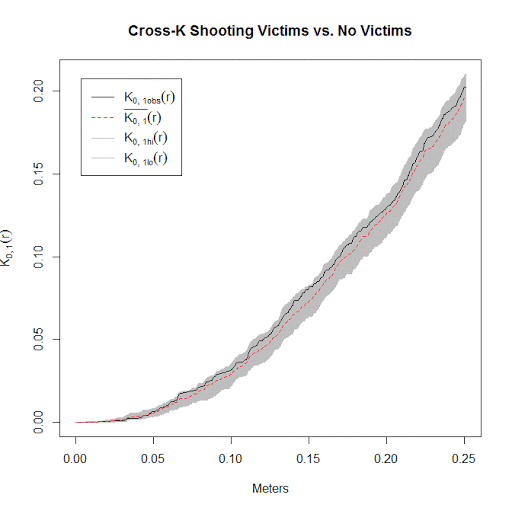I conducted this same analysis with actual shooting data in three separate cities that I have convenient access to the data. It is a hod podge of length, but City A and City B have around 100 shootings, and City C has around 500 shootings. In City A the observed line is very near the bottom, suggesting some evidence that shootings victims may be further apart than would be expected, but for most instances is within the 99% simulation band. (I can’t think of a theoretical reason why being spread apart would occur.) City B is pretty clearly within the simulation band, and City C’s observed pattern mirrors the mean of the simulation bands almost exactly. Since City C has the largest sample, I think this is pretty good evidence that shootings with a person hit are spatially random conditional on where shootings occur.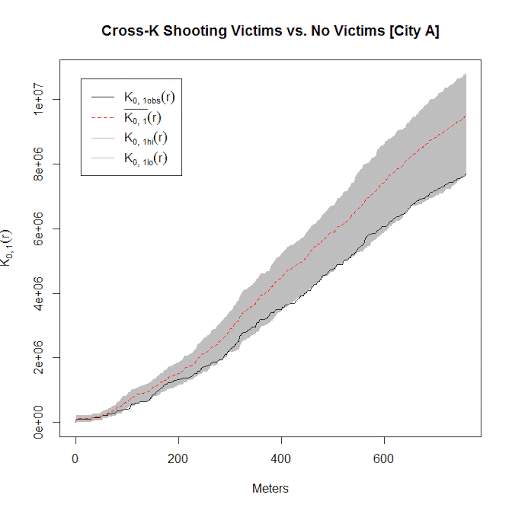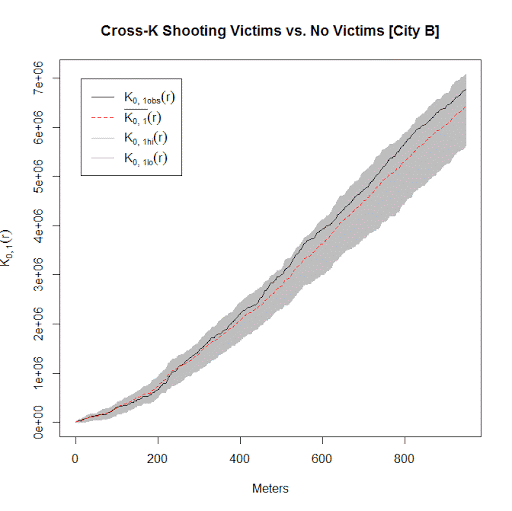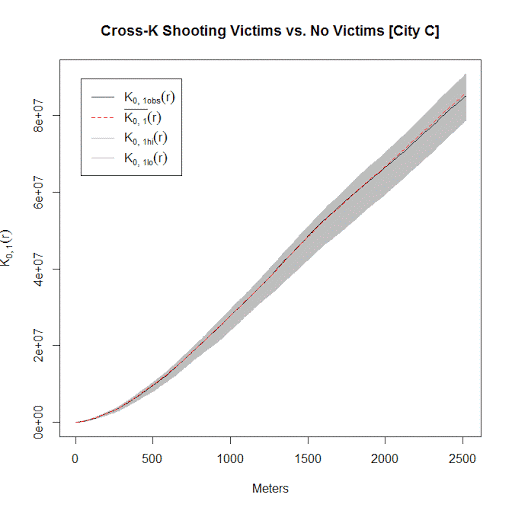Long story short, when conducting Ripley’s K with crime data, the default way to generate the simulation envelopes for the statistics are not appropriate given how crime data is recorded. I show here one way to account for that in generating simulation envelopes.

# One sided line buffers in R using rgeos

I’ve started to do more geographic data manipulation in R, and part of the reason I do blog posts is for self-reference, so I figured I would share some of the geographic functions I have been working on.

The other day on StackOverflow there was a question that asked how to do one sided buffers in R. The question was closed (and the linked duplicate is closed as well), so I post my response here.

The workflow I describe to make one sided buffers is in a nutshell

• expand the original polyline into a very small area by using a normal buffer, calls this Buf0
• do a normal two sided buffer on the original polyline, without square or rounded ends, call this Buf1
• take the geographic difference between Buf1 and Buf0, which basically splits the original buffer into two parts, and then return them as two separate spatial objects

Here is a simple example.

``````library(rgeos)
library(sp)

TwoBuf <- function(line,width,minEx){
Buf0 <- gBuffer(line,width=minEx,capStyle="SQUARE")
Buf1 <- gBuffer(line,width=width,capStyle="FLAT")
return(disaggregate(gDifference(Buf1,Buf0)))
}

Squig <- readWKT("LINESTRING(0 0, 0.2 0.1, 0.3 0.6, 0.4 0.1, 1 1)") #Orig
TortBuf <- TwoBuf(line=Squig,width=0.05,minEx=0.0001)
plot(TortBuf, col=c('red','blue'))  #First object on left, second on right``````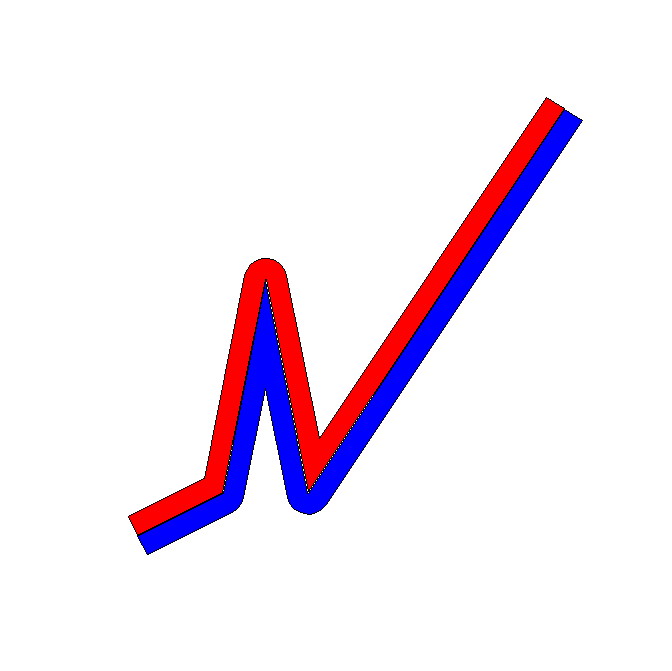If you imagine travelling along the polyline, which in this example goes from left to right, this is how I know the first red polygon is the left hand and the blue is the right hand side buffer. (To pick a specific one, you can subset like `TortBuf` or `TortBuf`.)

If we reverse the line string, the order will subsequently be reversed.

``````SquigR <- readWKT("LINESTRING(1 1, 0.4 0.1, 0.3 0.6, 0.2 0.1, 0 0)") #Reversed
TortBuf <- TwoBuf(line=SquigR,width=0.05,minEx=0.0001)
plot(TortBuf, col=c('red','blue'))  #Again first object on left, second on right``````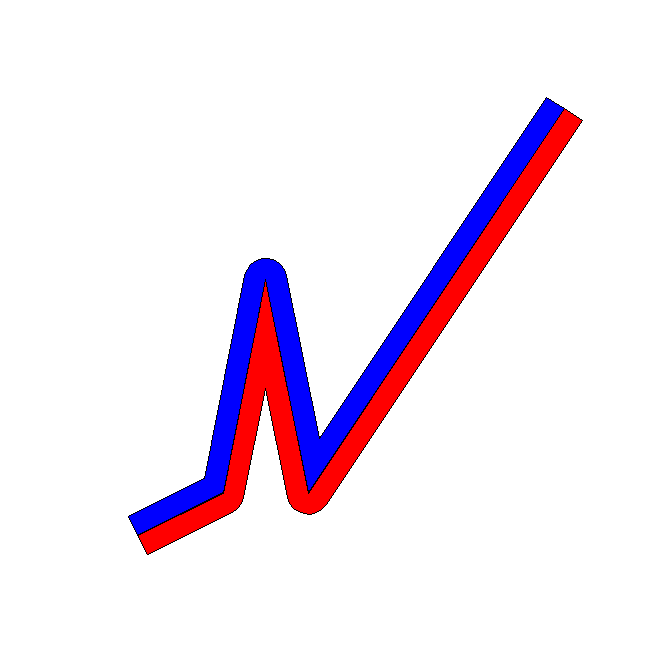Examples of south to north and north to south work the same as well.

``````SquigN <- readWKT("LINESTRING(0 0, 0 1)") #South to North
TortBuf <- TwoBuf(line=SquigN,width=0.05,minEx=0.0001)
plot(TortBuf, col=c('red','blue'))  #Again first object on left, second on right

SquigS <- readWKT("LINESTRING(0 1, 0 0)") #North to South
TortBuf <- TwoBuf(line=SquigS,width=0.05,minEx=0.0001)
plot(TortBuf, col=c('red','blue'))  #Again first object on left, second on right``````

One example in which this procedure does not work is if the polyline creates other polygons.

``````Square <- readWKT("LINESTRING(0 0, 1 0, 1 1, 0 1, 0 0)") #Square
TortBuf <- TwoBuf(line=Square,width=0.05,minEx=0.0001)
plot(TortBuf, col=c('red','blue','green'))

#Switch the direction
SquareR <- readWKT("LINESTRING(0 0, 0 1, 1 1, 1 0, 0 0)") #Square Reversed
TortBuf <- TwoBuf(line=SquareR,width=0.05,minEx=0.0001)
plot(TortBuf, col=c('red','blue','green'))                #Still the same order``````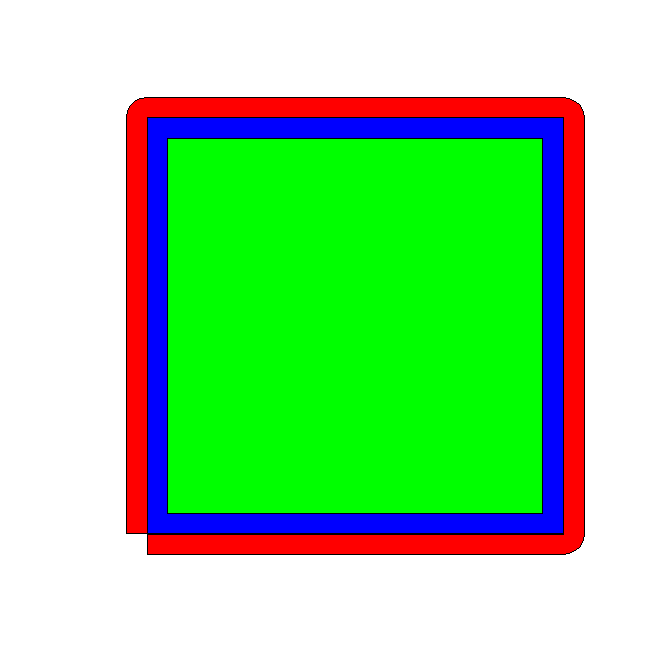This messes up the order as well. If you know that your polyline is actually a polygon you can do a positive and negative buffer to get the desired effect of interest. If I have a need to expand this to multipart polylines I will post an update, but I have some other buffer functions I may share in the mean time.

# Some plots to go with group based trajectory models in R

On my prior post on estimating group based trajectory models in R using the crimCV package I received a comment asking about how to plot the trajectories. The crimCV model object has a base plot object, but here I show how to extract those model predictions as well as some other functions. Many of these plots are illustrated in my paper for crime trajectories at micro places in Albany (forthcoming in the Journal of Quantitative Criminology). First we are going to load the `crimCV` and the `ggplot2` package, and then I have a set of four helper functions which I will describe in more detail in a minute. So run this R code first.

``````library(crimCV)
library(ggplot2)

long_traj <- function(model,data){
df <- data.frame(data)
vars <- names(df)
prob <- model['gwt'] #posterior probabilities
df\$GMax <- apply(prob\$gwt,1,which.max) #which group # is the max
df\$PMax <- apply(prob\$gwt,1,max)       #probability in max group
df\$Ord <- 1:dim(df)                 #Order of the original data
prob <- data.frame(prob\$gwt)
names(prob) <- paste0("G",1:dim(prob)) #Group probabilities are G1, G2, etc.
longD <- reshape(data.frame(df,prob), varying = vars, v.names = "y",
timevar = "x", times = 1:length(vars),
direction = "long") #Reshape to long format, time is x, y is original count data
return(longD)                        #GMax is the classified group, PMax is the probability in that group
}

weighted_means <- function(model,long_data){
G_names <- paste0("G",1:model\$ng)
G <- long_data[,G_names]
W <- G*long_data\$y                                    #Multiple weights by original count var
Agg <- aggregate(W,by=list(x=long_data\$x),FUN="sum")  #then sum those products
mass <- colSums(model\$gwt)                            #to get average divide by total mass of the weight
for (i in 1:model\$ng){
Agg[,i+1] <- Agg[,i+1]/mass[i]
}
long_weight <- reshape(Agg, varying=G_names, v.names="w_mean",
timevar = "Group", times = 1:model\$ng,
direction = "long")           #reshape to long
return(long_weight)
}

pred_means <- function(model){
prob <- model\$prob               #these are the model predicted means
Xb <- model\$X %*% model\$beta     #see getAnywhere(plot.dmZIPt), near copy
lambda <- exp(Xb)                #just returns data frame in long format
p <- exp(-model\$tau * t(Xb))
p <- t(p)
p <- p/(1 + p)
mu <- (1 - p) * lambda
t <- 1:nrow(mu)
myDF <- data.frame(x=t,mu)
long_pred <- reshape(myDF, varying=paste0("X",1:model\$ng), v.names="pred_mean",
timevar = "Group", times = 1:model\$ng, direction = "long")
return(long_pred)
}

#Note, if you estimate a ZIP model instead of the ZIP-tau model
#use this function instead of pred_means
pred_means_Nt <- function(model){
prob <- model\$prob               #these are the model predicted means
Xb <- model\$X %*% model\$beta     #see getAnywhere(plot.dmZIP), near copy
lambda <- exp(Xb)                #just returns data frame in long format
Zg <- model\$Z %*% model\$gamma
p <- exp(Zg)
p <- p/(1 + p)
mu <- (1 - p) * lambda
t <- 1:nrow(mu)
myDF <- data.frame(x=t,mu)
long_pred <- reshape(myDF, varying=paste0("X",1:model\$ng), v.names="pred_mean",
timevar = "Group", times = 1:model\$ng, direction = "long")
return(long_pred)
}

occ <- function(long_data){
subdata <- subset(long_data,x==1)
agg <- aggregate(subdata\$PMax,by=list(group=subdata\$GMax),FUN="mean")
names(agg) <- "AvePP" #average posterior probabilites
agg\$Freq <- as.data.frame(table(subdata\$GMax))[,2]
n <- agg\$AvePP/(1 - agg\$AvePP)
p <- agg\$Freq/sum(agg\$Freq)
d <- p/(1-p)
agg\$OCC <- n/d #odds of correct classification
agg\$ClassProp <- p #observed classification proportion
#predicted classification proportion
agg\$PredProp <- colSums(as.matrix(subdata[,grep("^[G][0-9]", names(subdata), value=TRUE)]))/sum(agg\$Freq)
#Jeff Ward said I should be using PredProb instead of Class prop for OCC
agg\$occ_pp <- n/ (agg\$PredProp/(1-agg\$PredProp))
return(agg)
}
``````

Now we can just use the data in the crimCV package to run through an example of a few different types of plots. First lets load in the `TO1adj` data, estimate the group based model, and make our base plot.

``````data(TO1adj)
plot(out1)``````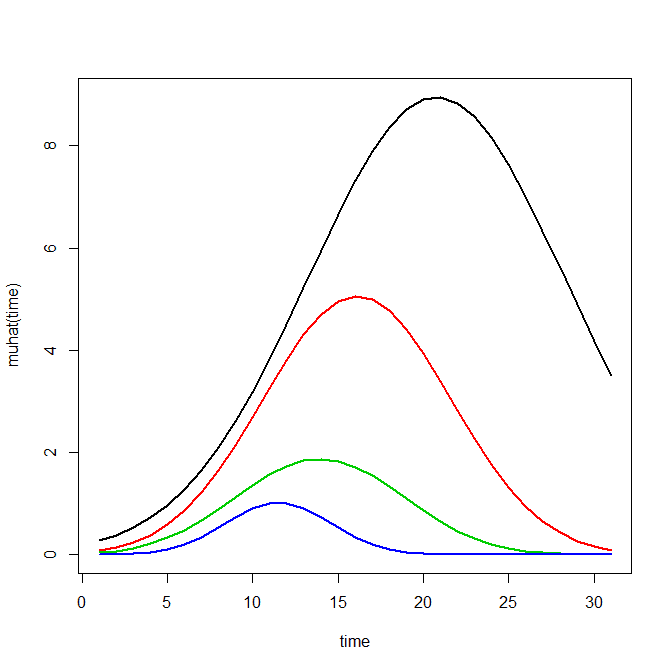Now most effort seems to be spent on using model selection criteria to pick the number of groups, what may be called relative model comparisons. Once you pick the number of groups though, you should still be concerned with how well the model replicates the data at hand, e.g. absolute model comparisons. The graphs that follow help assess this. First we will use our helper functions to make three new objects. The first function, `long_traj`, takes the original model object, `out1`, as well as the original matrix data used to estimate the model, `TO1adj`. The second function, `weighted_means`, takes the original model object and then the newly created long_data `longD`. The third function, `pred_means`, just takes the model output and generates a data frame in wide format for plotting (it is the same underlying code for plotting the model).

``````longD <- long_traj(model=out1,data=TO1adj)
x <- weighted_means(model=out1,long_data=longD)
pred <- pred_means(model=out1)``````

We can subsequently use the long data `longD` to plot the individual trajectories faceted by their assigned groups. I have an answer on cross validated that shows how effective this small multiple design idea can be to help disentangle complicated plots.

``````#plot of individual trajectories in small multiples by group
p <- ggplot(data=longD, aes(x=x,y=y,group=Ord)) + geom_line(alpha = 0.1) + facet_wrap(~GMax)
p ``````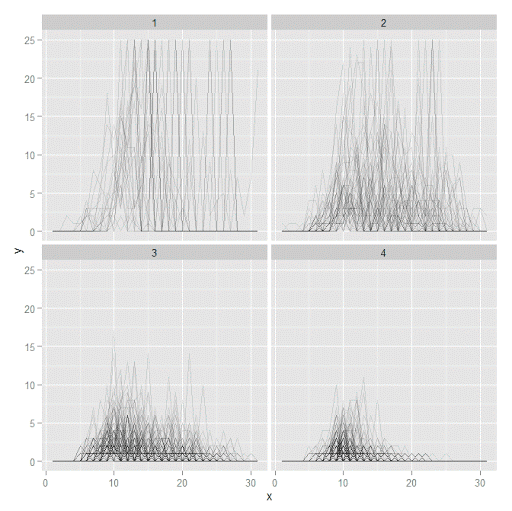Plotting the individual trajectories can show how well they fit the predicted model, as well as if there are any outliers. You could get more fancy with jittering (helpful since there is so much overlap in the low counts) but just plotting with a high transparency helps quite abit. This second graph plots the predicted means along with the weighted means. What the `weighted_means` function does is use the posterior probabilities of groups, and then calculates the observed group averages per time point using the posterior probabilities as the weights.

``````#plot of predicted values + weighted means
p2 <- ggplot() + geom_line(data=pred, aes(x=x,y=pred_mean,col=as.factor(Group))) +
geom_line(data=x, aes(x=x,y=w_mean,col=as.factor(Group))) +
geom_point(data=x, aes(x=x,y=w_mean,col=as.factor(Group)))
p2``````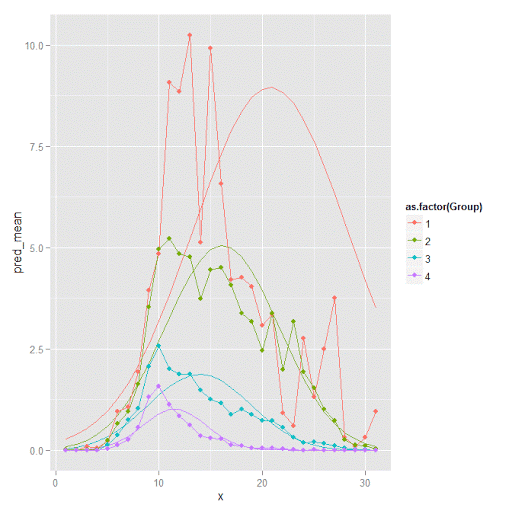Here you can see that the estimated trajectories are not a very good fit to the data. Pretty much eash series has a peak before the predicted curve, and all of the series except for 2 don’t look like very good candidates for polynomial curves.

It ends up that often the weighted means are very nearly equivalent to the unweighted means (just aggregating means based on the classified group). In this example the predicted values are a colored line, the weighted means are a colored line with superimposed points, and the non-weighted means are just a black line.

``````#predictions, weighted means, and non-weighted means
nonw_means <- aggregate(longD\$y,by=list(Group=longD\$GMax,x=longD\$x),FUN="mean")
names(nonw_means) <- "y"

p3 <- p2 + geom_line(data=nonw_means, aes(x=x,y=y), col='black') + facet_wrap(~Group)
p3``````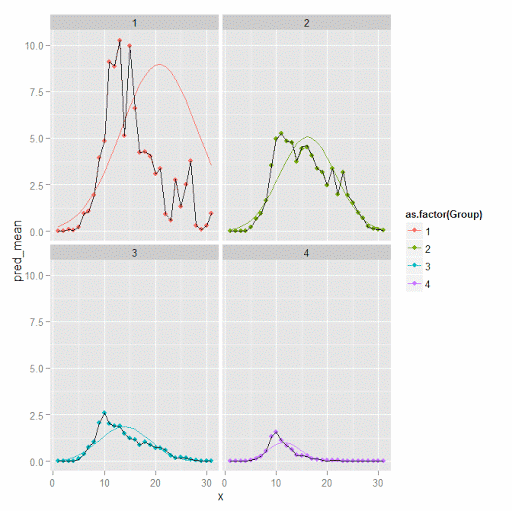You can see the non-weighted means are almost exactly the same as the weighted ones. For group 3 you typically need to go to the hundredths to see a difference.

``````#check out how close
nonw_means[nonw_means\$Group==3,'y'] -  x[x\$Group==3,'w_mean']``````

You can subsequently superimpose the predicted group means over the individual trajectories as well.

``````#superimpose predicted over ind trajectories
pred\$GMax <- pred\$Group
p4 <- ggplot() + geom_line(data=pred, aes(x=x,y=pred_mean), col='red') +
geom_line(data=longD, aes(x=x,y=y,group=Ord), alpha = 0.1) + facet_wrap(~GMax)
p4``````Two types of absolute fit measures I’ve seen advocated in the past are the average maximum posterior probability per group and the odds of correct classification. The `occ` function calculates these numbers given two vectors (one of the max probabilities and the other of the group classifications). We can get this info from our long data by just selecting a subset from one time period. Here the output at the console shows that we have quite large average posterior probabilities as well as high odds of correct classification. (Also updated to included the observed classified proportions and the predicted proportions based on the posterior probabilities. Again, these all show very good model fit.) Update: Jeff Ward sent me a note saying I should be using the predicted proportion in each group for the occ calculation, not the assigned proportion based on the max. post. prob. So I have updated to include the occ_pp column for this, but left the old occ column in as a paper trail of my mistake.

``````occ(longD)
#  group     AvePP Freq        OCC  ClassProp   PredProp     occ_pp
#1     1 0.9880945   23 1281.00444 0.06084656 0.06298397 1234.71607
#2     2 0.9522450   35  195.41430 0.09259259 0.09005342  201.48650
#3     3 0.9567524   94   66.83877 0.24867725 0.24936266   66.59424
#4     4 0.9844708  226   42.63727 0.59788360 0.59759995   42.68760
``````

A plot to accompany this though is a jittered dot plot showing the maximum posterior probability per group. You can here that groups 3 and 4 are more fuzzy, whereas 1 and 2 mostly have very high probabilities of group assignment.

``````#plot of maximum posterior probabilities
subD <- longD[x==1,]
p5 <- ggplot(data=subD, aes(x=as.factor(GMax),y=PMax)) + geom_point(position = "jitter", alpha = 0.2)
p5``````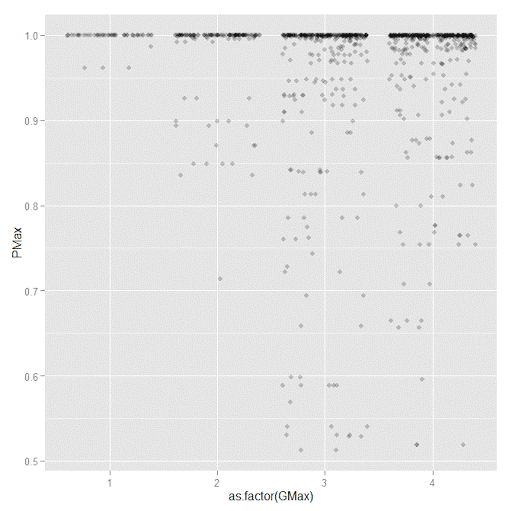Remember that these latent class models are fuzzy classifiers. That is each point has a probability of belonging to each group. A scatterplot matrix of the individual probabilities will show how well the groups are separated. Perfect separation into groups will result in points hugging along the border of the graph, and points in the middle suggest ambiguity in the class assignment. You can see here that each group closer in number has more probability swapping between them.

``````#scatterplot matrix
library(GGally)
sm <- ggpairs(data=subD, columns=4:7)
sm``````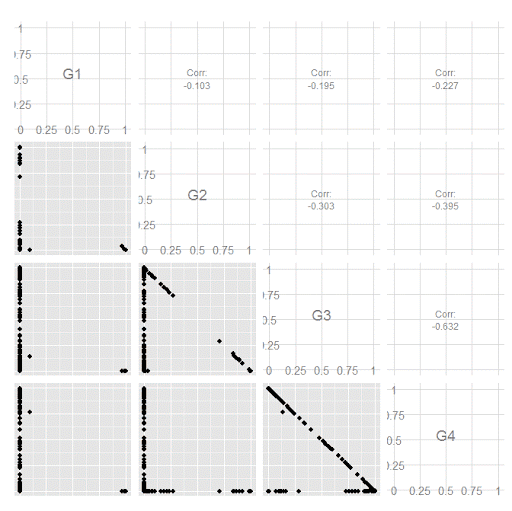And the last time series plot I have used previously is a stacked area chart.

``````#stacked area chart
nonw_sum <- aggregate(longD\$y,by=list(Group=longD\$GMax,x=longD\$x),FUN="sum")
names(nonw_sum) <- "y"
p6 <- ggplot(data=nonw_sum, aes(x=x,y=y,fill=as.factor(Group))) + geom_area(position='stack')
p6``````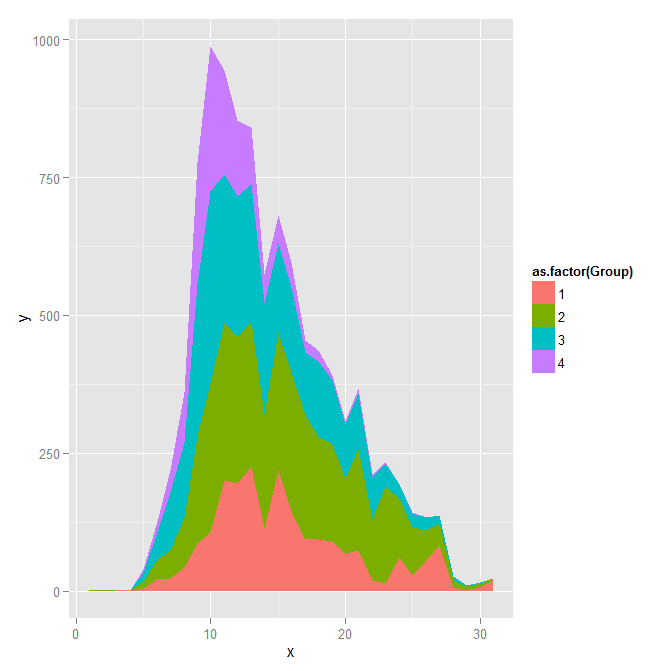I will have to put another post in the queue to talk about the spatial point pattern tests I used in that trajectory paper for the future as well.